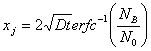# Formation of a pn Junction and Junction Depth Calculations

The distance from the surface, xj, at which the diffused impurity profile intersects the background concentration NB, is called the metallurgical junction xj. The net impurity concentration at xj is zero.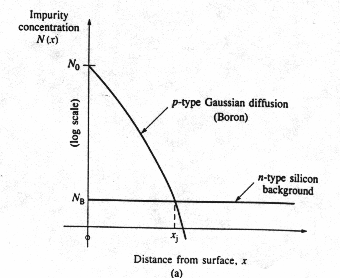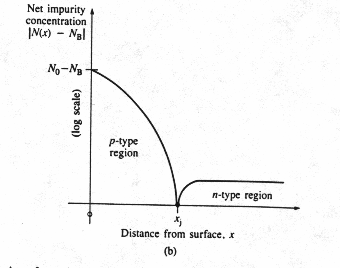Fig 4 Formation of a pn junction by diffusion (a) an example of a p-type Gaussian diffusion into a uniformly doped n-type wafer; (b) net impurity concentration in the wafer. The metallurgical junction occurs at the point x=xj, where the net concentration is zero. The material is converted to p-type to the left of xj and remains n-type to the right of xj. 

For Gaussian Distribution

Impurity concentration at distance x and time t, that is, N(x,t) is given by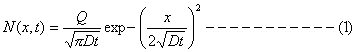We know that at junction depth, that is, x=xj, N(x,t)=NB, the background concentration. Therefore, we have,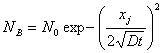at x= xj N(x,t) = NB

The junction depth is given by the following expression.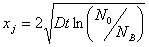For Complementary Error Function Distribution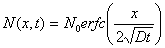At x=xj N(x,t)=NB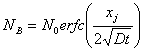The junction depth is given by the following expression.